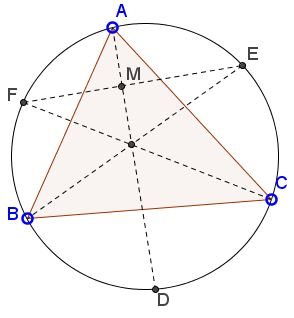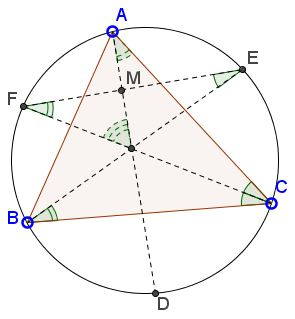Angle Bisectors On Circumcircle

What is this about? A Mathematical Droodle

ExplanationAngle Bisector

• Angle Bisector
• Angle Bisector Theorem
• All about angle bisectors
• Angle Bisectors in Ellipse
• Angle Bisectors in Ellipse II
• Angle Bisector in Equilateral Trapezoid
• Angle Bisector in Rectangle
• Property of Angle Bisectors
• Property of Angle Bisectors II
• A Property of Angle Bisectors III
• External Angle Bisectors
• Projections on Internal and External Angle Bisectors
• Angle Bisectors in a Quadrilateral - Cyclic and Otherwise
• Problem: Angle Bisectors in a Quadrilateral
• Triangle From Angle Bisectors
• Property of Internal Angle Bisector - Hubert Shutrick's PWW
• Angle Bisectors Cross Circumcircle
• For Equality Choose Angle Bisector
•The applet attempts to suggest the following problem [Tao, pp. 50-51]:

ABC is a triangle that is inscribed in a circle. The angle bisectors of A, B, C meet the circle in D, E, F, respectively. Show that AD is perpendicular to EF.We'll concentrate on ΔFIM.By a theorem of the inscribed angles,

∠IFM = ∠CFE = ∠CBE = ∠B/2.

By a the theorem of the secant angles (or with the help of the Exterior Angle Theorem),

∠FIM = ∠ACI + ∠CAI = ∠C/2 + ∠A/2.

It follows that in ΔFIM, angles at F and I add up to 90°:

∠A/2 + ∠B/2 + ∠C/2 = 180°/2 = 90°.

We conclude that the remaining angle at M is necessarily right.

References

1. T. Tao, Solving Mathematical Problems, Oxford University Press